# Engineering Mechanics - Force System Resultants

Exercise : Force System Resultants - General Questions
• Force System Resultants - General Questions
21.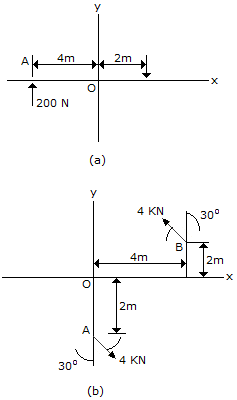Determine the magnitude and direction of the couple shown.

M = 22.6 kN-m CCW
M = 22.6 kN-m CW
M = 21.9 kN-m CCW
M = 21.9 kN-m CW
Explanation:
No answer description is available. Let's discuss.

22.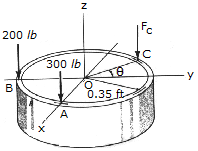Three parallel forces act on the rim of the tube. If it is required that the resultant force FR of the system have a line of action that coincides with the central z axis, determine the magnitude of FC and its locationon the rim. What is the magnitude of the resultant force FR?

Fc = 361 lb,= 56.3°, R = 861 lb
Fc = 500 lb,= 54.0°, R = 1000 lb
Fc = 500 lb,= 36.0°, R = 1000 lb
Fc = 361 lb,= 36.9°, R = 861 lb
Explanation:
No answer description is available. Let's discuss.

23.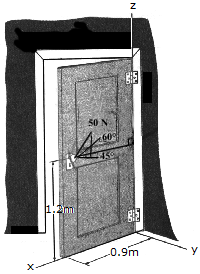A force of 50 N is applied to the handle of the door as shown. Determine the projection of the moment of this force about the hinged axis z. Neglect the size of the doorknob. Suggestion: Use a scaler analysis.

Mz = 15.9 N-m
Mz = -15.9 N-m
Mz = -27.6 N-m
Mz = 27.6 N-m
Explanation:
No answer description is available. Let's discuss.

24.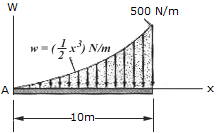The wind has blown sand over a platform such that the intensity of load can be approximated by the function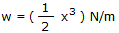. Simplify this distributed loading to a single concentrated force and specify the magnitude and location of the force measured from A.

R = 1250 N, x = 6.67 m
R = 2500 N, x = 6.67 m
R = 1250 N, x = 8 m
R = 2500 N, x = 8 m
Explanation:
No answer description is available. Let's discuss.

25.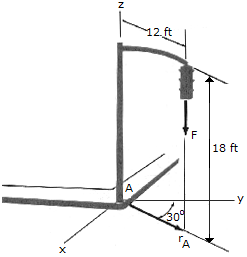The pole supports a 22-lb traffic light. Using Cartesian vectors, determine the moment of the weight of the traffic light about the base of the pole at A.

MA = 216k lb-ft
MA = (-132i-229j-216k) lb-ft
MA = (-229i+121j) lb-ft
MA = (-132i+229j) lb-ft